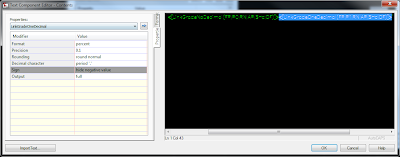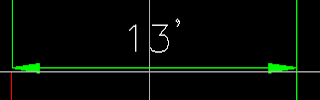## Wednesday, June 22, 2011

### Label Precision Blues

I was recently asked to create a label that was capable of showing either one decimal precision or zero. The label was for cross sections, and there are a lot of them so I didn't want to have to go through manually and change the label or code set style to get the correct precision. In order to get the desired results I used two expressions.

The first expression checks to see if the number is not a whole number. In order to do this I used some multiplication, subtraction and if then statements.

There are two evaluations evaluated in the above drawing. The first checks to see if the rounded value is 1, the second checks to see if it's 0. Each evaluation returns either a 1 for true or a 0 for false. Adding them the evaluations we can see if one of the cases is true. Since Civil 3D will evaluate a result of 1 as true and 0 as false.
The second expression is the same, except the return values are switched.

Then I added both expressions to the label to making sure that I hide any negative values.I then am able to use one label style to show two precisions since if one is true the other won't be shown and vice versa.

So this label:Is the same as this one: